There are no notes in Mattrab Library for this chapter yet.

1. At dawn and dusk, large amount of blue and violet light(shorter wavelength) has been scattered so, the light that is recieved by an observer is mostly of a longer wavelength and therefore appears to be red.

2. A lens is a piece of transparent glass bounded by two refracting surfaces which are usually spherical.

Two uses of the lens are:

1. It is used in the human spectacle.
2. It is used in scientific projects.

3.The different types of lens are:

Convex lens: A lens that is thick in the middle and thin at the edge is called a convex lens.

Concave lens: A lens that is thick at the edges but thin in the middle is called a concave lens.

4. a. Principal axis:
The line joining both the centers of curvature of a lens is called the principal axis.

b. Optical center:
The geometrical center of a lens is known as its optical center.

c. Center of curvature:
The center of the sphere from which the lens has been cut is called the center of curvature.

d. Focal length:
The distance between principle focus and optical center is called the focal length.

e. Far point:
The distance point from the eye seen clearly is called the far point.

f. Near point:
The nearest point up to which an object clearly is called near point.

g. Accommodation of an eye:
The ability of an eye to focus the image of objects at various distances on the retina by changing the focal length of the eye lens is called accommodation of an eye.

h. Range of vision:
The distance between the far point and the near point is called the range of vision.

5. The process of adjusting the distance between a lens and the screen so that the image becomes clear and distinct is called focusing.

Focusing is done to produce a sharp and clear image in the camera, microscope, telescope, etc.

6. a. at 2F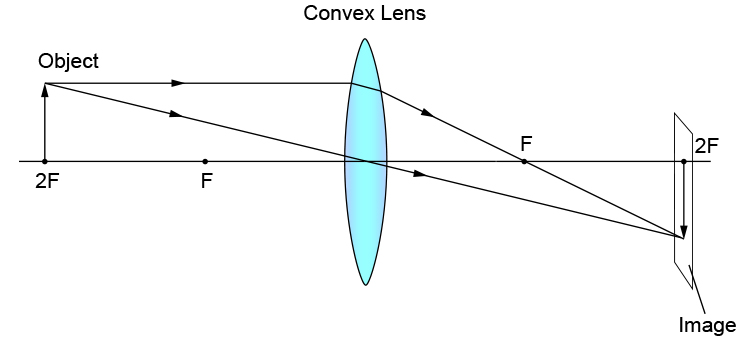Nature: Real and inverted
Position: 2F

b. Between 2F and F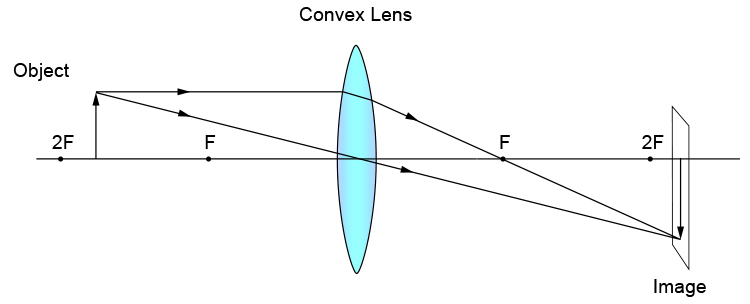Nature: Real and inverted
Position: Beyond 2F

c. Af F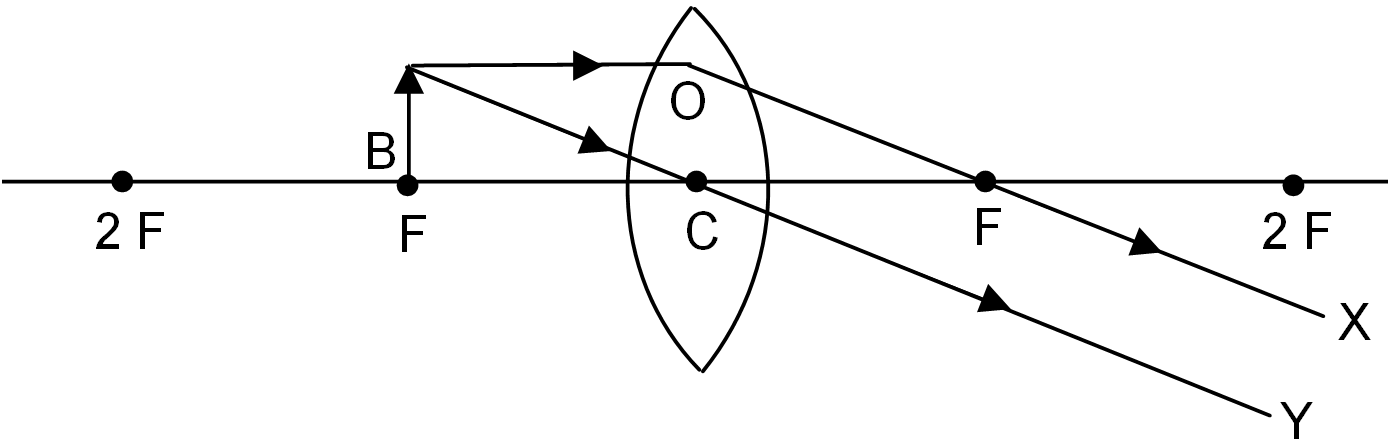Nature: Real and inverted
Position: At infinity

d. Between F and O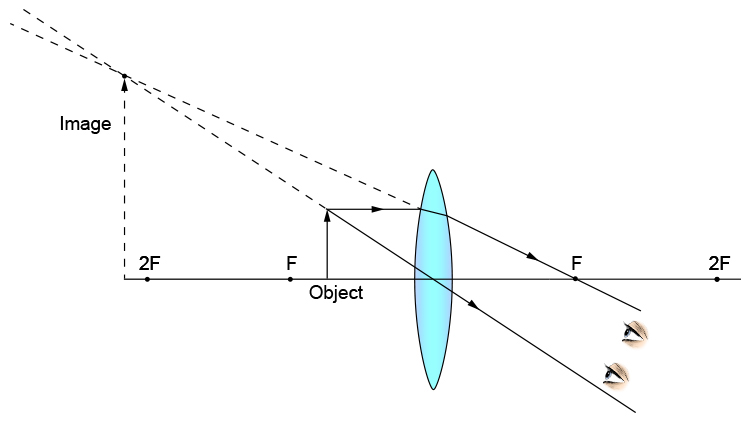Nature: Virtual and erect
Position: Beyond the object

e. Beyond 2F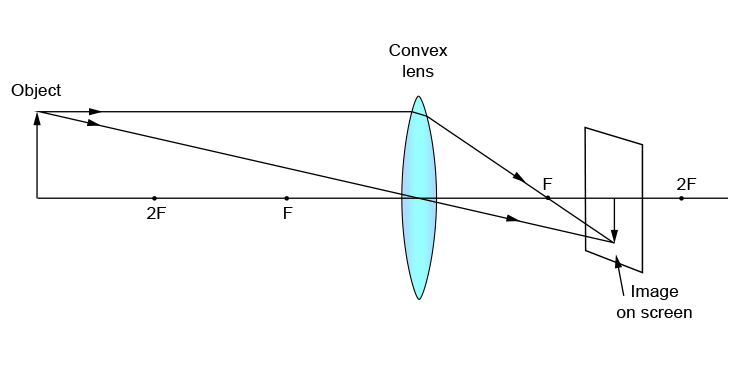Nature: Real and inverted
Position: Between F and 2F

7. When the object is placed between F and O of a convex lens, the lens forms an erect and enlarged image.

8.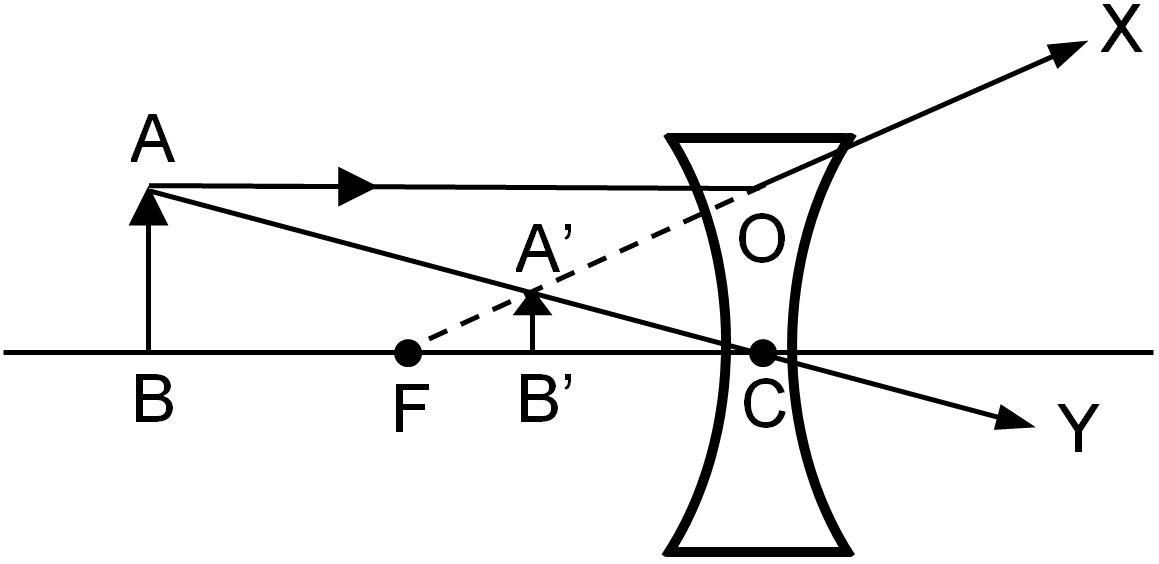Nature: Virtual and erect
Position: Between F and O

9. The power of the lens is defined as the reciprocal of its focal length in meter.
Its SI unite dioptre (D).

10. The ratio of the height of the image to the height of the object is called magnification.

As we know that, the instrument that is used to produce an image of an object with the help of a lens, is called an optical instrument. In the same way, the camera works on the basis of refraction or reflection of light. So, it is called an optical instrument.

11.12. a. Pupil
It controls the amount of light entering into the eye.

b. Iris
It is responsible for controlling the diameter and size of the pupil.

c. Cornea
It protects the eye and allows the light to pass.

d. Eye lens
It focuses the image changing the focal length.

e. Ciliary muscle
It helps in changing the focal length of the eye lens.

f. Retina
It sends the electric impulses to the brain through the optic nerve.

g. Optic nerve
It acts as the medium to transmit the impulses to and from the brain and retina.

Vitreous humor
It helps the eyeball to retain its shape.

13. The distant point from the eye which can be seen clearly is called the far point of the eye. The normal range of vision of the human eye is about 25 cm.

14. Myopia is the defect of vision in which a person can see a nearby object but cannot see distant objects.

Its causes are:

1. Due to the shorting of focal length high converging power of the eye lens.
2. Due to the elongation of eyeballs.

Its remedy is:

1. It can be corrected by using a concave lens of suitable focal length.15. Hypermetropia is a defect of vision in which a person can see distant objects clearly but cannot see nearby objects.

Its causes are:

1. Due to the increase of focal length low converging power of the eye lens.
2. Due to the contraction of the eyeball.

Its remedy is:

1. It can be corrected by using a convex lens of a suitable focal lenght.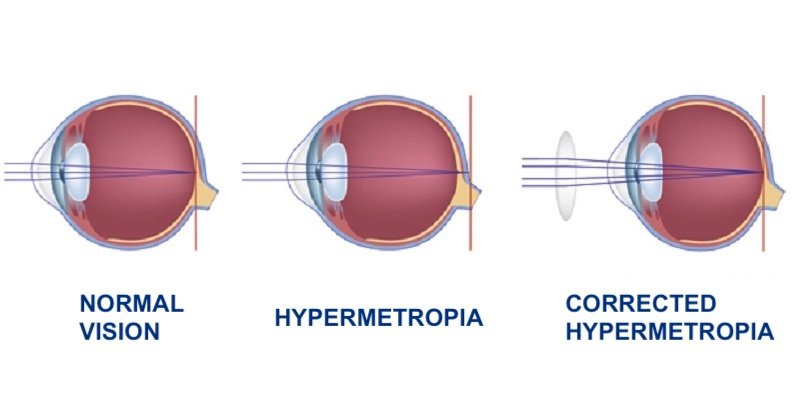16. As we know that, a lens that is thick in the middle and thin as the edges is a convex lens. It converges the parallel beam of light at a point after refraction. So, it is called a converging lens.

17. As we know that a lens that is thin in the middle and thick at the edges is called a concave lens. It diverges the parallel beam of light to different points after refraction. It has a virtual focus. So, it is called a diverging lens.

18. As we know, hypermetropia is the defect of vision in which a person can see distant objects but cannot see nearby objects. While using a convex lens, the rays of the light coming from a nearby object are first converged by the convex lens and by the eye lens. Due to the combined converging action of both lenses, the image is formed at the retina. So, a convex lens is used in spectacles to remove hypermetropia.

19. As we know, myopia is the defect of vision in which a person can see nearby objects but cannot see distant objects. While using a concave lens, the rays of the light coming from a nearby object are first diverged by the concave lens and by the eye lens. Due to the combined diverging action of both lenses, the image is formed at the retina. So, a concave lens is used in spectacles to remove myopia.

20. a. Convex and concave lens.

 Convex Concave It is thick in the middle and thin at the edges. It is thin in the middle and thick at the edges. It converges the parallel beam of light. It diverges the parallel beam of light. It has a real focus. It has a virtual focus. It has +ve power. It has -ve power.

b. Virtual image and real image.

 Virtual Real It cannot be cast on the screen. I can be cast on the screen. It is always erect. It is always inverted. It is formed by the apparent intersection of rays at a point. It is formed by the actual intersection of rays at a point.

c. Myopia and hypermetropia.

 Myopia Hypermetropia It is the defect of vision in which a person can see nearby objects but cannot see distant objects. It is the defect of vision in which a person can see distant objects but cannot see nearby objects. It is also called short-sightedness. It is also called long-sightedness. It is corrected by using a concave lens. It is corrected by using a convex lens.

d. Far point and near point.

 Far point Near point The distant point from the eye which can be seen clearly is called the far point. The nearest point which an eye can see the object clearly is called the near point. The far point is at infinity for a normal eye. The near point is at 25 cm for a normal eye.

There are no MCQs in Mattrab Library for this chapter yet.